# ISEE Middle Level Math : How to find median

## Example Questions

### Example Question #51 : How To Find Median

Find the median in this set of numbers: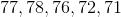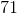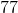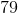Explanation:

First order the numbers from least to greatest: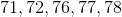Then, identify the middle number: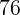Answer:### Example Question #52 : How To Find Median

Find the median for this set of numbers: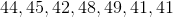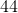None of theseExplanation:

First, order the numbers from least to greatest: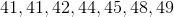Then, identify the middle number. Since there are seven numbers, the middle will be the fourth number from either end:.

Answer:### Example Question #53 : How To Find Median

Find the median for this set of numbers: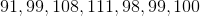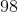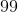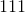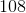Explanation:

First order the numbers from least to greatest: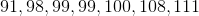Then identify the middle number. Since there are seven numbers, the middle will be the fourth number from either side:.

Answer:### Example Question #54 : How To Find Median

Find the median in this set of numbers: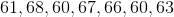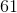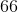Explanation:

First order the numbers from least to greatest: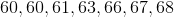Then, identify the middle number:Answer:### Example Question #55 : How To Find Median

Find the median for this set of numbers: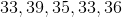None of theseExplanation:

First order the numbers from least to greatest: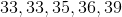Then identify the middle number:Answer:### Example Question #56 : How To Find Median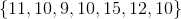Find the median of the data set above.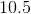Explanation:

To find the median of a data set, you have to put the set in order from least to greatest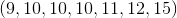. Then cross out one number from each side until you are left with the middle number, or the median. In this case, it's 10.

### Example Question #57 : How To Find Median

Find the median in this set of number: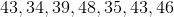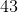Explanation:

First order the numbers from least to greatest: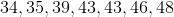Then, identify the middle number:Answer:### Example Question #58 : How To Find Median

Find the median for this set of numbers: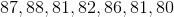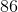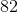Explanation:

First, order the numbers from least to greatest: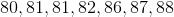Then, identify the middle number. Since there are seven numbers total, the middle will be the fourth number from either end:Answer:### Example Question #59 : How To Find Median

Find the median in this set of numbers: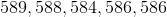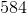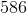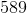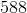Explanation:

First order the numbers from least to greatest: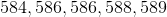Identify the middle number:Answer:### Example Question #60 : How To Find Median

Find the median in this set of numbers: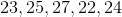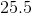Explanation:

First order the numbers from least to greatest: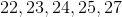Then, identify the middle number:Answer:### All ISEE Middle Level Math Resources